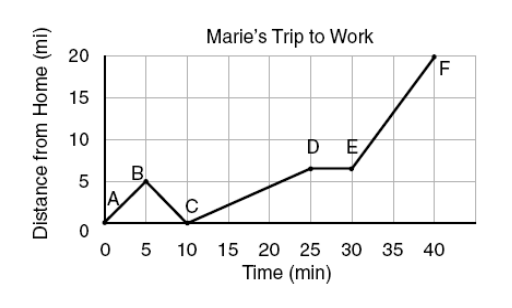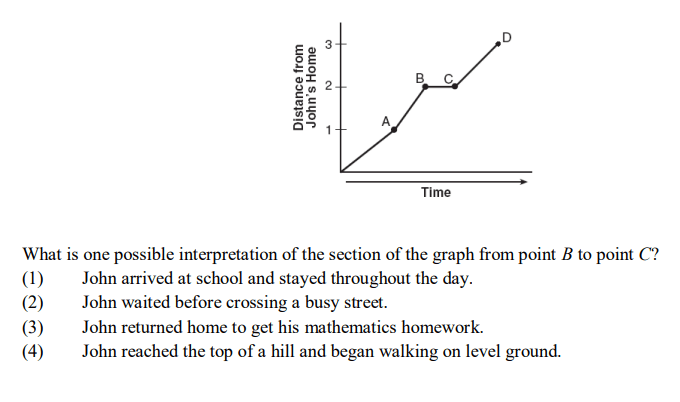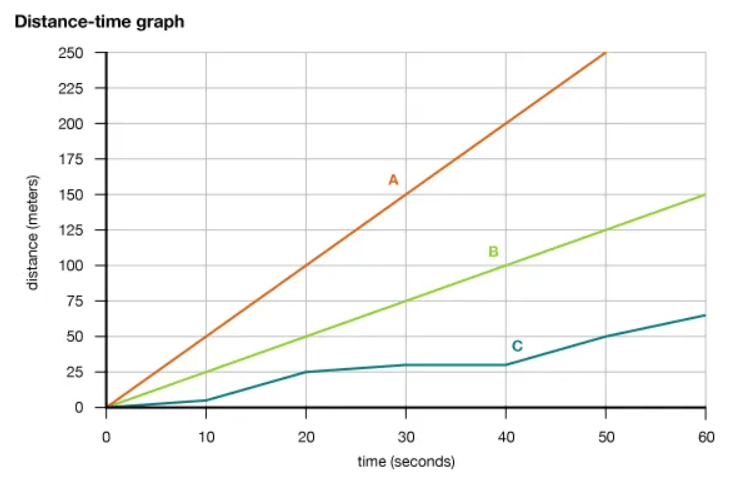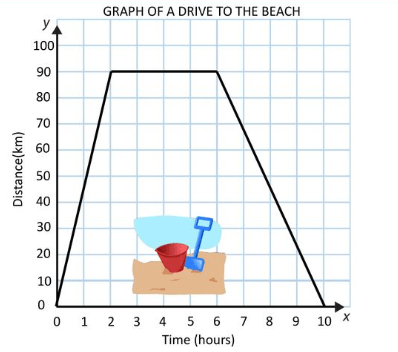Speed/Velocity
Calculations
Acceleration
Equations and Units
Distance & Time Graphs
100
The rate at which an object moves a certain distance.
What is speed?
100

The velocity of a speed that ran 321 meters in 70 seconds.

What is 4.6 m/s?

100

What is a change in velocity or direction?

acceleration

100

What are meters used to measure?

What is the unit for distance?

100

Below is a graph of Maria's trip to work. At what point did she realize she forgot something and had to go back home?B

200
How fast an object moves over a certain distance in a direction.
What is velocity?
200

The distance an object travels if the speed is 26 m/s and the time is 23 seconds.

What is 598 meters?

200

What is negative acceleration?

When an object slows down.

200

The equation used to solve for distance.

What is distance = speed x time?

200

John walks to school every day on a straight path. Below is John's walk.2

300

How do you calculate speed?

distance divided by time

300

What is the time it takes an object to travel 198 meters at a speed of 34 m/s.

What is 5.8 seconds?

300

An object that is not accelerating is moving in a ____________.

What is moving at a constant speed and direction.

300

The equation used to solve for time.

What is time = distance/speed?

300

Which line represents the fasted person?A

400

An object that is motionless on a distance-time graph is represented by a ____________.

What is a flat horizontal line.

400

The speed of an object that travels 23 meters in 18 seconds.

What is 1.28 m/s?

400
Speeding up, slowing down, or changing directions.
What are examples of acceleration?
400

The SI unit of measurement for speed?

What is Meters per Second (m/s)

400

The graph shows a family's trip to the beach. When did the family drive faster: to the beach or on their way home?To the beach.

500

The steeper the line on a distance-time graph, the _________ the speed.

faster

500

What is the distance an object travels if going at a speed of 8 m/s in 28 seconds.

What is 224 meters?

500

A given point that helps to determine if an object has moved.

What is a reference point?

500

The equation used to solve for Average Speed.

What is Average Speed = total distance / total time?

500

The graph shows a family's trip to the beach. How long did the family stay at the beach?4 hours

Click to zoom